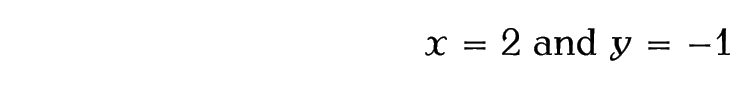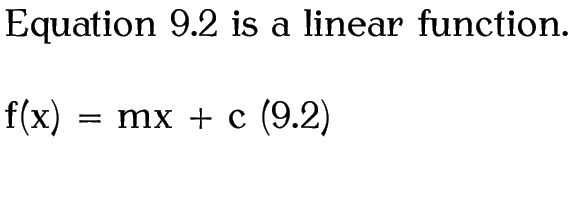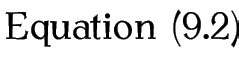# 9.2 Displayed Mathematics

One-line unnumbered displayed mathematics can be created using:

$<maths>$

where <maths> is the mathematics to be displayed.

Example:

A linear function is a function of the form
$y = mx + c$

Output:Don't use the displaymath environment or $$...$$ . Use $and$ with the amsmath package.

The equation environment provides something similar to , except that the equation is numbered. Modifying the above example:

A linear function is a function of the form
\begin{equation}
y = mx + c
\end{equation}

results in the following output:Normal text can be inserted into the equation using

\text{<text>}

which is provided by the amsmath package.

Example:

$x = 2 \text{ and } y = -1$

results in the following output:[Re-using an equation]Recall from §5.5. Cross-Referencing that we can cross-reference most things that LaTeX automatically numbers using \ref and \label. Equations can be cross-referenced in the same way:

Equation~\ref{eqn:linear} is a linear function.
\begin{equation}
\label{eqn:linear}
f(x) = mx + c
\end{equation}Equation numbers are usually given in parentheses, which can be done using:

Equation~(\ref{eqn:linear})

The amsmath package provides a convenient short cut:

\eqref{<label>}

So the above can be written as:

Equation~\eqref{eqn:linear}Note:

Both the equation environment and $...$ are only designed for one line of maths. Therefore you must not have any line breaks or paragraph breaks within them. The following will cause an error:

\begin{equation}

f(x) = mx + c

\end{equation}
Either remove the blank lines or comment them out:
\begin{equation}
%

f(x) = mx + c
%

\end{equation}

This book is also available as A4 PDF or 12.8cm x 9.6cm PDF or paperback (ISBN 978-1-909440-00-5).

© 2012 Dickimaw Books. "Dickimaw", "Dickimaw Books" and the Dickimaw parrot logo are trademarks. The Dickimaw parrot was painted by Magdalene Pritchett.